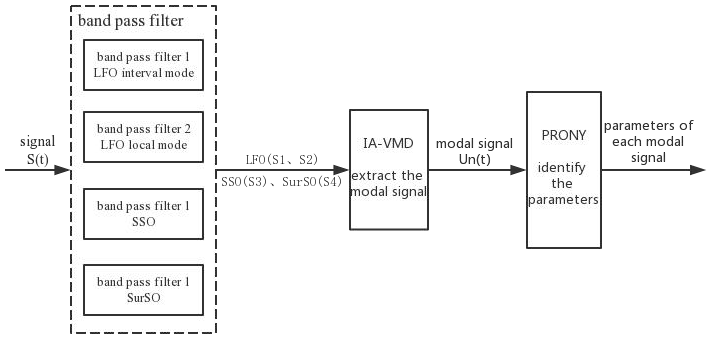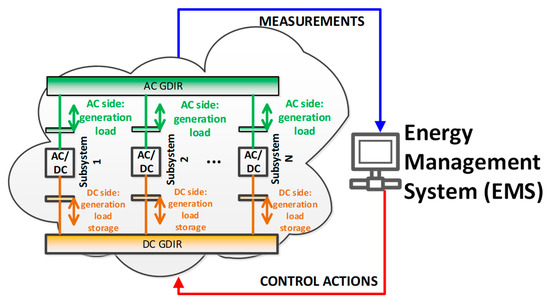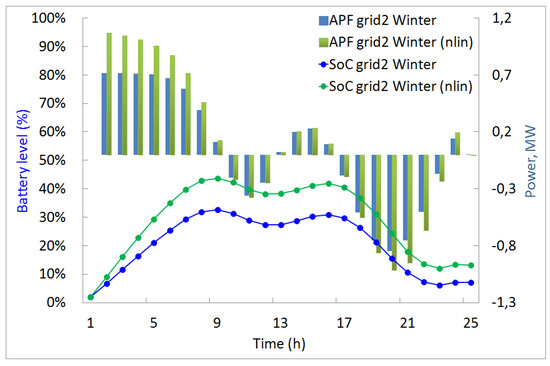## 基于风电系统非线性动态建模的短期功率预测与储能控制研究

#### Project Leads: Prof. RAS Denis Sidorov (ESI SB RAS) and Prof. Fang Liu (CSU)

Wind power systems are characterized by generation variability. The project deals with the integration of wind energy systems using new energy storage control algorithms, as well as forecasting models of electric load and wind generation. Effective storage control models are proposed, which are expressed in terms of nonlinear systems of evolutionary Volterra nonlinear integral equations of the first kind that allow to take into account the efficiency and state-of-change reduction and also able to effectively determine the active mass of battery degradation. To solve these nonlinear systems, effective numerical methods based on a modified Newton-Kantorovich iterative process and spline collocation are proposed. A general convergence theorem for the method is proved. An estimate for the rate of convergence is obtained. The theoretical basement of a methodology and software have been proposed for storage control and for wind speed and power forecasting using fuzzy Takagi-Sugeno models and electrical loads forecasting. Forecasting models employ the deep learning based on convolutional-based bidirectional gated recurrent unit and tested on electric load and air pollution datasets. The project will improve the efficiency of AC/DC micro grids with storage system in the nature protection zone of Lake Baikal.

### 1. Nonlinear Systems of Volterra Equations with Piecewise Smooth Kernels: Numerical Solution and Application for Power Systems OperationThe evolutionary integral dynamical models of storage systems are addressed. Such models are based on systems of weakly regular nonlinear Volterra integral equations with piecewise smooth kernels. These equations can have non-unique solutions that depend on free parameters. The objective of this paper was two-fold. First, the iterative numerical method based on the modified Newton–Kantorovich iterative process is proposed for a solution of the nonlinear systems of such weakly regular Volterra equations. Second, the proposed numerical method was tested both on synthetic examples and real world problems related to the dynamic analysis of microgrids with energy storage systems.

### 2. Mode Identification of Broad-band Oscillation Signal Based on Improved VMD MethodOscillation is one of the most important factors that affect the safety and stability of power system. In a power system, there may be multiple types of oscillation signal occurring at the same time. In this paper, a method based on improved variational modal decomposition (VMD) algorithm is proposed to identify the mode of broad-band oscillation signal. First, different types of oscillation are separated from broad-band oscillation signal by band pass filter. And then the modal signals are extracted from the filtered signals by improved VMD method. Finally, the parameters of the modal signals are identified by prony algorithm. The simulation shows that the method can extract the modal signals from broad-band signal and identify the parameters accurately

### 3. Toward Zero-Emission Hybrid AC/DC Power Systems with Renewable Energy Sources and Storages: A Case Study from Lake Baikal RegionTourism development in ecologically vulnerable areas like the lake Baikal region in Eastern Siberia is a challenging problem. To this end, the dynamical models of AC/DC hybrid isolated power system consisting of four power grids with renewable generation units and energy storage systems are proposed using the advanced methods based on deep reinforcement learning and integral equations. First, the wind and solar irradiance potential of several sites on the lake Baikal’s banks is analyzed as well as the electric load as a function of the climatic conditions. The optimal selection of the energy storage system components is supported in online mode. The approach is justified using the retrospective meteorological datasets. Such a formulation will allow us to develop a number of valuable recommendations related to the optimal control of several autonomous AC/DC hybrid power systems with different structures, equipment composition and kind of AC or DC current. Developed approach provides the valuable information at different stages of AC/DC hybrid power systems projects development with stand-alone hybrid solar-wind power generation systems.

### 4. Recurrent Neural Networks Application to Forecasting with Two Cases: Load and PollutionForecasting problems exist widely in our life. Its purpose is to enable decision makers to make effective responses to future changes. The traditional prediction methods based on probability and statistics cannot guarantee the accuracy of multivariable dynamic prediction under the background of high randomness and big data. In recent years, with the improvement of hardware computing ability and the large-scale increase of training data, deep learning has been widely applied in the field of forecasting. This paper focuses on the analysis of the application of recurrent neural networks (RNN), an advanced algorithm in deep learning, in the forecasting task. The forecasting models based on long short-term memory (LSTM) and gated recurrent unit (GRU) were established respectively, and the real data of power load and air pollution were verified. Compared with traditional machine learning algorithms, the simulation proves the superiority of the forecasting model based on RNN.

### 5. Solvability and Bifurcation of Solutions of Nonlinear Equations with Fredholm Operator

The necessary and sufficient conditions of existence of the nonlinear operator equations’ branches of solutions in the neighbourhood of branching points are derived. The approach is based on the reduction of the nonlinear operator equations to finite-dimensional problems. Methods of nonlinear functional analysis, integral equations, spectral theory based on index of Kronecker-Poincaré, Morse-Conley index, power geometry and other methods are employed. Proposed methodology enables justification of the theorems on existence of bifurcation points and bifurcation sets in the nonstandard models. Formulated theorems are constructive. For a certain smoothness of the nonlinear operator, the asymptotic behaviour of the solutions is analysed in the neighbourhood of the branch points and uniformly converging iterative schemes with a choice of the uniformization parameter enables the comprehensive analysis of the problems details. General theorems and effectiveness of the proposed methods are illustrated on the nonlinear integral equations.

### 7. Control of accuracy on Taylor-collocation method to solve the weakly regular Volterra integral equations of the first kind by using the CESTAC method

Finding the optimal parameters and functions of iterative methods is among the main problems of the Numerical Analysis. For this aim, a technique of the stochastic arithmetic (SA) is used to control of accuracy on Taylor-collocation method for solving first kind weakly regular integral equations (IEs). Thus, the CESTAC method is applied and instead of usual mathematical software the CADNA library is used. Also, the convergence theorem of presented method is illustrated. In order to apply the CESTAC method we will prove a theorem that it will be our licence to use the new termination criterion instead of traditional absolute error. By using this theorem we can show that number of common significant digits (NCSDs) between two successive approximations are almost equal to NCSDs between exact and numerical solution. Finally, some examples are solved by using the Taylor-collocation method based on the CESTAC method. Several tables of numerical solutions based on the both arithmetics are presented. Comparison between number of iterations are demonstrated by using the floating point arithmetic (FPA) for different values of epsilon.

### 8. Machine Learning for Energy Systems